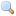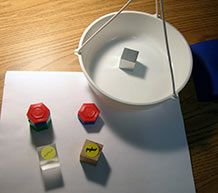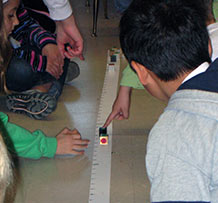Investigating Standard Measures 2:

# How much do the cubes weigh in grams?

## Plan Investigating Standard Measures 2

Students have been thinking about the cubes and their relative weights for a while. In this investigation, they see those relationships expressed in grams. While the activity is simple — just weigh and compare — students’ thinking about weight becomes more sophisticated.

### Formative Assessment

Do students know the weights of the 8 cubes in grams? Do they understand why they'll use grams as the standard unit of weight?

Available online at inquiryproject.terc.edu

Students weigh the cubes in grams and place them on the weight line. As they study the array, they focus on two embedded ideas: the additive nature of weight and the continuous nature of weight. They also have another opportunity to ponder why these same–sized cubes have such different weights.

By the end of the investigation, students will be able to describe more explicitly how the weight of one cube compares to another.

### Learning Goals

• to determine the gram weight of the materials cubes
• to use a weight line to compare the weights of objects
• to consider the additive nature of weight
• to appreciate the continuous nature of weights
Sequence of experiences
1. Ask the question All Class 5 Mins
2. Weigh the cubes in grams Small Groups 15 Mins
3. Add the cubes to the weight line All Class 5 Mins
4. Make meaning All Class 15 Mins

### Materials and Preparation

For the class:
• Post the investigation question in a place where all students can see it.
• Make a class table on a whiteboard or flip chart for recording the gram weights of the cubes; an example is shown in Step 2.
• the plastic weight line
• 1 index card labeled “Grams”
• 2 or more sets of materials cubes (each student needs one cube to weigh)
• 2 cubes that have been cut in half
For each group:
• 1 gram weight set
• 2 pan balances

### Notebook Pages

Formative Assessment

## Do students know the weights of the 8 cubes in grams? Do they understand why they'll use grams as the standard unit of weight?

Look at notebook entries to find evidence that the student has an accurate record of the weight of each cube in grams. You'll also find evidence of their understanding of the rationale for using grams to weigh things.Enlarge (2) Notebook Pages

Use these criteria to interpret student work:

• The cubes should weigh approximately:
Pine 7g, Oak 13g, Nylon 18g, Acrylic 19g, PVC 22, Aluminum 44g, Steel 128g and Copper 147g. Humidity will affect the weights of pine and oak, and the (in)accuracy of the balances may cause the measurements of all cubes to vary by one or two grams.
• Rationales for using grams:
this is the unit used by scientists around the world, like paper clips, they are light enough to discriminate between objects that are very close in weight.

Next steps might include selecting another small object, estimating its weight in grams, and weighing it.

All Class 05 MinsShow students a set of materials cubes and tell them they will weigh the cubes in grams, just as they weighed the classroom objects. Remind students that grams are the units scientists use.

This will be the students’ first opportunity to discover how the weights of the cubes compare in terms of grams. Point students’ attention to the investigation question:

“How much do the cubes weigh in grams?”

Let students know that more than one student will weigh each type of cube. Scientists want other scientists to try the same measurement or experiment, to see if they get the same result. This is one way that scientists learn if their work is accurate, or if they have made a mistake.

• Why do scientists want other scientists to try the same measurement or experiment?

Give every student one cube to weigh. As you hand each student a cube, ask them to think about or predict how much their cube weighs in grams.

## 2. Measure and record the gram weights

Small Groups 15 Mins Notebook

Students work in small groups to weigh their cubes and record the weight in grams in their science notebooks. As they finish, students enter their results in the class table.

Cube Material Weight #1 (grams) Weight #2 (grams) Weight #3 (grams) Final Weight (grams)
Pine
Oak
Nylon
Acrylic
PVC
Aluminum
Steel
Copper

Table Note: Classes with more than 16 students will need part of a third set of cubes in order for every student to weigh a cube. Classes with more than 24 students will need a forth column.

Review the results in the class table. If cubes of the same type have weight differences of a full gram or more, ask students to swap the cubes in question and weigh them one more time. Finally, have students record in their science notebooks the agreed-upon “Final Weight” of the full set of cubes.

## 3. Add the cubes to the weight line

All Class 5 MinsHave students gather at the weight line with their cubes, and draw attention to the unit label: “Grams.” Invite one or two students at a time to place their cubes on the line, taking their weight data from the class table or from their notebooks. Allow multiple cubes of the same material on the weight line to give everyone a sense of completion for this part of the work.

## 4. Make meaning

All Class 15 Mins

### Purpose of the discussion

The purpose of this discussion is to bring forward the idea that weight is a property that can be increased in small or large increments.

Students can see just “how much heavier” some cubes are than others; they can even express the weight differences with some precision.

### Engage students in the focus question

How much weight do I have to add to cube A to make it the same weight as cube B?

Draw students’ attention to the weight difference between pairs of cubes by asking a series of questions cast in this form:

• How much weight do I need to add to cube A to make it the same weight as cube B?

This is a different way of asking the question, “How much heavier … ?” It avoids focusing attention on the individual weights of the cubes and instead highlights the size of the difference between them. It also emphasizes the additive nature of weight. Start by asking students about cubes that are fairly close together:

• How much would I have to add to the acrylic cube to make it weigh the same as the PVC cube?
• How much would I have to add to the pine cube to make it weigh the same as the oak cube?